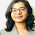## Monday, 4 March 2013

### CALCULATE FACTORIAL OF VERY BIG NUMBERS THROUGH A SIMPLE C PROGRAM

Here is one more interesting program that enables you to calculate the factorial of very massive numbers such as 500. And even you can calculate the sum of all the digits of this massive number.
Have a look at this program and enjoy.
This program runs perfectly well in turbo cpp.

Enjoy programming:)

/*******************************************************************************/

#include<stdio.h>
#include<conio.h>
#include<iostream.h>
#include<time.h>
#define max 5000
void multiply(long int *,long int);
void factorial(long int *,long int);

int main()
{
clrscr();
cout<<"PROGRAM TO CALCULATE FACTORIAL OF A NUMBER";
cout<<"\nENTER THE NUMBER\n";
long int num;
cin>>num;

long int a[max];
for(long int i=0;i<max;i++)
a[i]=0;

factorial(a,num);

clrscr();

//PRINTING THE FINAL ARRAY...:):):)
cout<<"THE FACTORIAL OF "<<num<<" is "<<endl<<endl;
long int flag=0;

int ans=0;
for(i=0;i<max;i++)
{
if(flag||a[i]!=0)
{
flag=1;
cout<<a[i];
ans=ans+a[i];
}
}

cout<<endl<<endl<<"the sum of all digits is: "<<ans;

getch();
return 1;
}

void factorial(long int *a,long int n)
{
long int lavish;
long int num=n;
lavish=n;
for(long int i=max-1;i>=0&&n;i--)
{
a[i]=n%10;
n=n/10;
}

for(i=2;i<(lavish);i++)
{
multiply(a,num-1);
num=num-1;

}
}

void multiply(long int *a,long int n)
{

for(long int i=0;i<max;i++)
a[i]=a[i]*n;

for(i=max-1;i>0;i--)
{
a[i-1]=a[i-1]+(a[i]/10);
a[i]=a[i]%10;
}
}

/*****************************************************************************/

For some simple factorial calculating functions refer the following links :-

#### 2 comments:

1.This comment has been removed by the author.

2.Gud efforts.. Dis way v cn get out of d box programs.